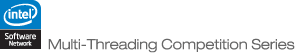JOINProblem Statement

Problem: Distance

### Problem Statement

The execution of your program in this problem will take place in two stages. In the first stage, you will be given a large undirected graph, and will have 30 seconds to perform any processing you desire. In the second stage, you will be queried about pairs of points in the graph, and must determine the distance between them. In the second stage, you will only be allowed 2 seconds of processing time, for 10000 pairs of points.

Scoring will be based on how how much time you use in the second stage, combined with the accuracy the distances you return. The accuracy will be computed using the root mean squared error (RMSE) of your returned distances. In other words, for each distance, we will compute the error from the correct distance, and square it. We then take the average of these squared errors, and finally take the square root of this average. We will then add time to this error, where time is your stage 2 execution time, measured in seconds. Your final score will be 10*tests minus the sum of your individual scores, where tests is the number of test cases. If you score over 10 on any test case, or you fail any test case (exceed time, crash, or provide an invalid return), it will be treated as if you scored 10.

Each graph except the examples will have one million nodes. The nodes will be numbered from 0 to 999,999. A value D will then be chosen between 5 and 20, along with a real value a between 0 and 1. For each node, D edges will be created. When creating an edge from node i, it will go to node j (where j does not equal i) with probability proportional to ((di,j)-a-(di,j+1)-a), where di,j is the minimum of |i-j| and N-|i-j|. This may generate duplicate edges, so there may end up being fewer than N*D edges in the graph given to you. Finally, the nodes will be randomly permuted so that the numbering used to generate the graph is lost.

You should write a method process that takes a String[], representing the graph. Element i of the input is a space delimited list of nodes that i is connected to. For conciseness, if j appears on i's list, i will not neccesarily appear on j's list (though it may), though the edges are undirected. The method query will take two int[]s, and should return a double[] of the same length. Element i of the returned double[] should contain the distance between u[i] and v[i].

### Definition

 Class: Distance Method: process Parameters: String[] Returns: int Method signature: int process(String[] g) Method: query Parameters: int[], int[] Returns: double[] Method signature: double[] query(int[] u, int[] v) (be sure your methods are public)

### Notes

-There will be a path in the graph between all queried pairs of nodes.
-While some of the examples are smaller graphs with fewer queries, all of the test cases will be on one million nodes, with 10,000 queries.
-While the actual path lengths are clearly integers, you may find it useful to predict fractional values. For example, if you are not sure whether the path length between two nodes is 8 or 9, you might predict 8.5.
-The memory limit is 1024M and the thread limit is 32 (including the primary thread).
-java.util.Random is used for all random number generation.
-There are 20 non-example test cases.

### Examples

0)

`This test case has 10 nodes.  D = 5 and a = 0.6294993817708533.  There are 10 queries.`
 Download Each of the first N lines represents a single node. Each line starts with an integer K, and is followed by K other integers representing K edges. For example if line 0 were "2 83 76", it would indicate that there were edges from node 0 to nodes 83 and 76. Following these N lines, are a number of lines containing two integers -- the queries. All test case files are formatted the same way. Note that the file format is slightly different from the input format. The graph in the file for this test cases is represented by ```2 8 6 4 5 0 4 2 3 0 6 9 3 7 8 0 3 5 1 7 3 9 4 1 4 4 0 7 9 4 4 1 5 8 4 3 7 1 2 3 2 5 1 ``` On the other hand, the String[] input is: ```{"8 6", "5 0 4 2", "0 6 9", "7 8 0", "5 1 7", "9 4 1", "4 0 7 9", "4 1 5 8", "3 7 1 2", "2 5 1"} ```
1)

`This test case has 100 nodes.  D = 20 and a = 0.4809864913712196.  There are 100 queries.`
2)

`This test case has 1000 nodes.  D = 16 and a = 0.05128392223198952.  There are 1000 queries.`
3)

`This test case has 10000 nodes.  D = 10 and a = 0.3403916445508408.  There are 10000 queries.`
4)

`This test case has 100000 nodes.  D = 7 and a = 0.9106890605104496.  There are 10000 queries.`
5)

`This test case has 1000000 nodes.  D = 5 and a = 0.5126586954905619.  There are 10000 queries.`
6)

`This test case has 1000000 nodes.  D = 18 and a = 0.08295611145017068.  There are 10000 queries.`
7)

`This test case has 1000000 nodes.  D = 12 and a = 0.37206384867018316.  There are 10000 queries.`
`This test case has 1000000 nodes.  D = 9 and a = 0.942361279530953.  There are 10000 queries.`
`This test case has 1000000 nodes.  D = 7 and a = 0.7938483742301582.  There are 10000 queries.`